# 9.5 Encoder

### What is an Encoder?

An encoder is a circuit that changes a set of signals into a code. Let’s begin making a 2-to-1 line encoder truth table by reversing the 1-to-2 decoder truth table.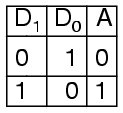This truth table is a little short. A complete truth table would be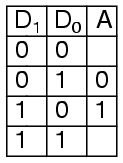One question we need to answer is what to do with those other inputs? Do we ignore them? Do we have them generate an additional error output? In many circuits, this problem is solved by adding sequential logic in order to know not just what input is active but also which order the inputs became active.

### Encoder Design Applications

A more useful application of combinational encoder design is a binary to 7-segment encoder. The seven segments are given according to:Our truth table is: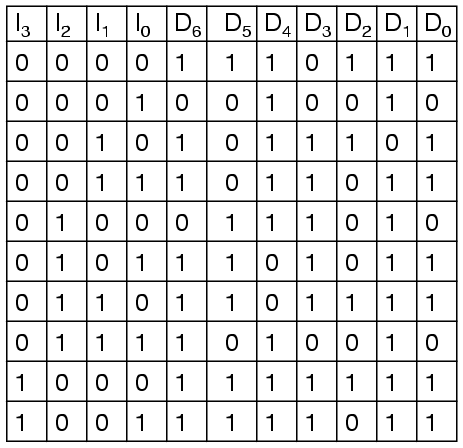Deciding what to do with the remaining six entries of the truth table is easier with this circuit. This circuit should not be expected to encode an undefined combination of inputs, so we can leave them as “don’t care” when we design the circuit. The equations were simplified with Karnaugh maps.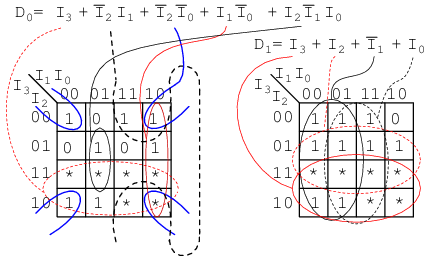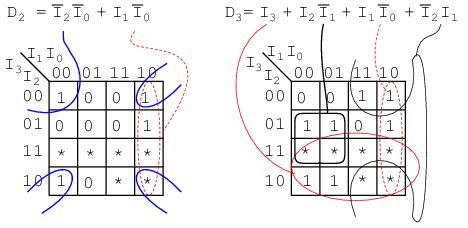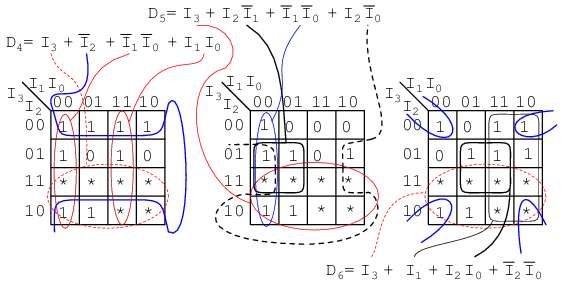### Equation Collection Summary

The collection of equations is summarized here: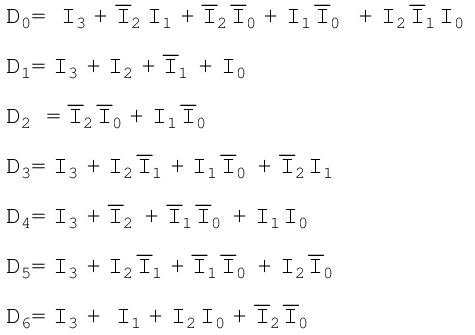### The Resulting Circuit Diagram

The circuit is: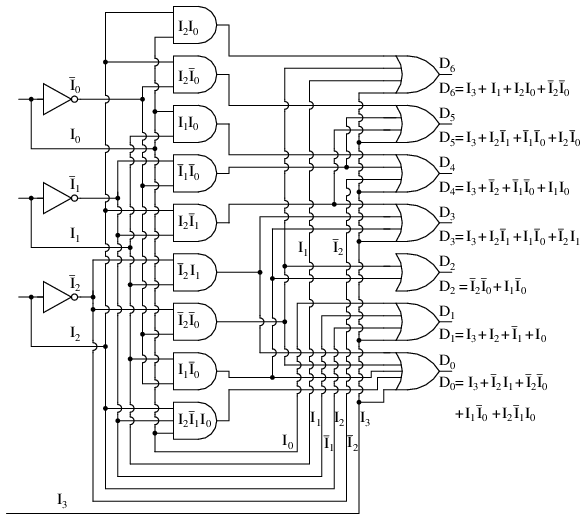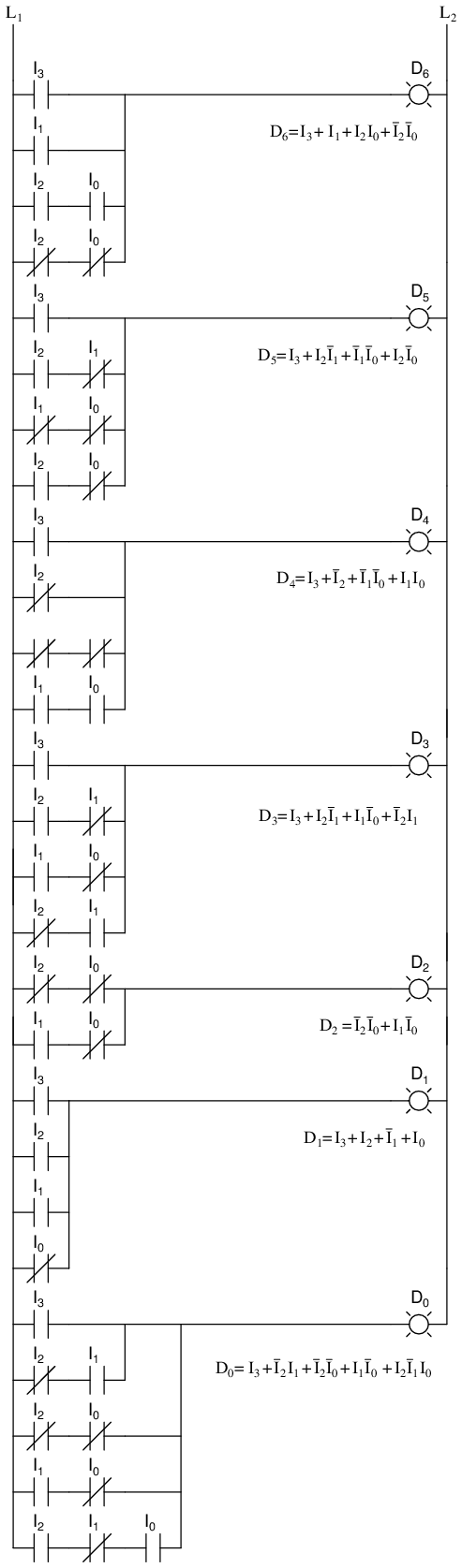Back to Main Index of Book